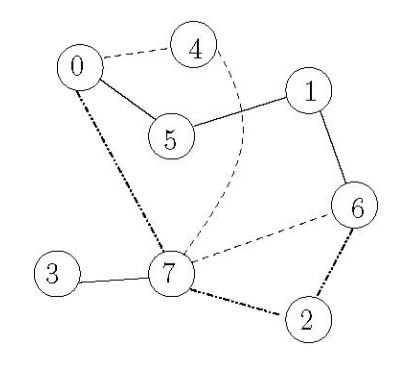시간 제한메모리 제한제출정답맞힌 사람정답 비율
1 초 128 MB36000.000%

## 문제

Given a connected computer network (bidirectional communication) we want to find two different nodes u and v such that we can maximize the congestion between u and v with a continuously sent virus being sent between the pair. We define the congestion level as the maximum number of edge-disjoint paths between nodes u and v.

For example, the network shown in the following figure has three different paths between nodes 0 and 6 such that each edge used is only part of one connecting path. Note that two paths are allowed to go through the same node, such as node 7. No other pair of nodes provides a higher congestion level.## 입력

We will be given a sequence of connected computer networks each with n nodes, n ≤ 40, labeled {0, 1,...,n − 1}. The last input case will be followed by a network of n =0 nodes, which should not be processed.

The specification for a computer network will be as follows: the first line contains a single non-negative value n, denoting the number of nodes. This is then followed by n lines of integers, separated by spaces, denoting the neighbors (zero indexed) of each node. Expect up to 2000 test cases.

## 출력

For each input case output one integer on a line by itself denoting the maximum congestion level possible for some pair of its network nodes.

## 예제 입력 1

8
4 5 7
5 6
6 7
7
0 7
0 1
1 2 7
0 2 3 4 6
4
1 2
0 2
3 0 1
2
0


## 예제 출력 1

3
2